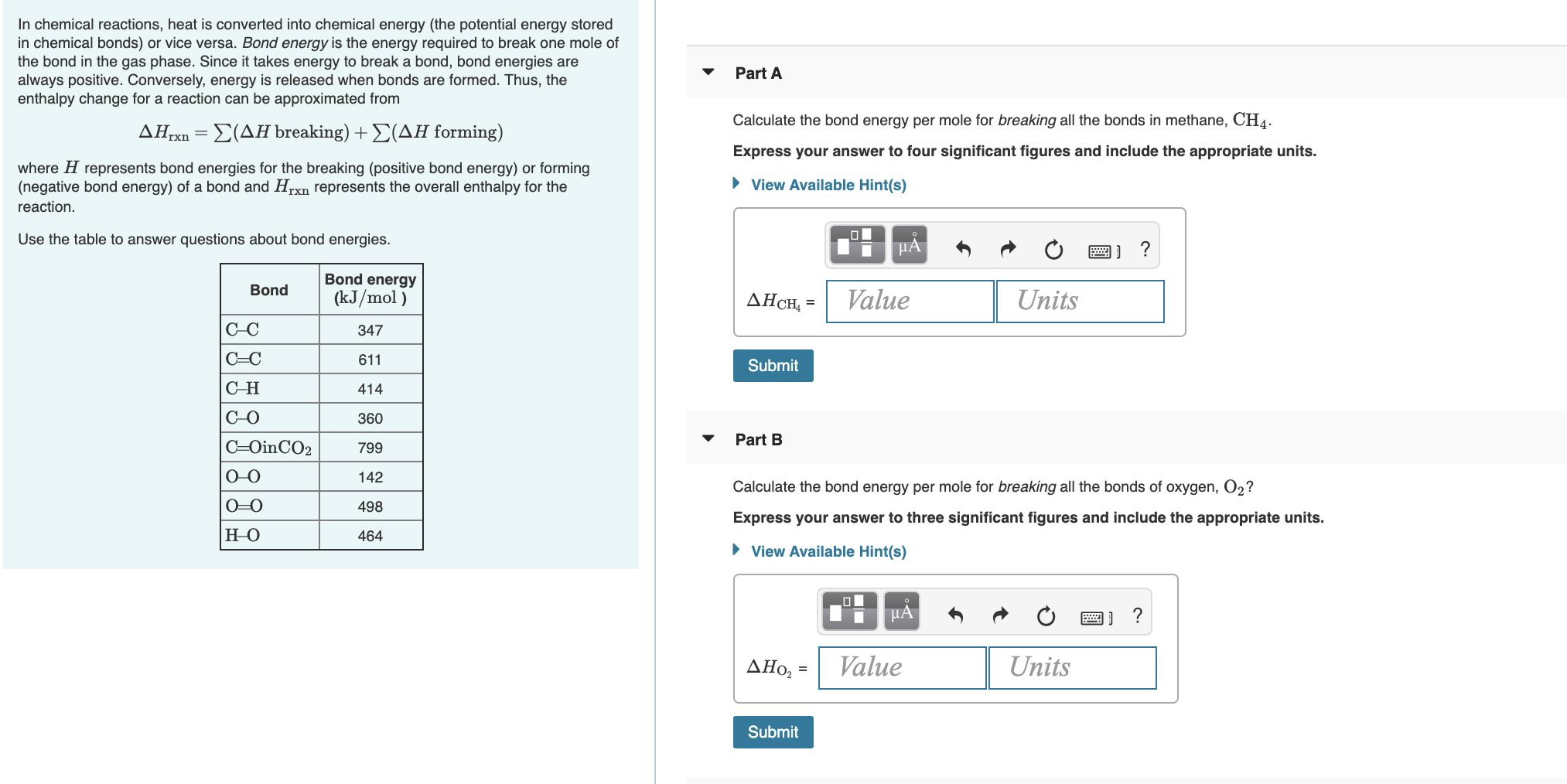# In chemical reactions, heat is converted into chemical energy (the potential energy storedin chemical bonds) or vice versa. Bond energy is the energy required to break one mole ofthe bond in the gas phase. Since it takes energy to break a bond, bond energies arealways positive. Conversely, energy is released when bonds are formed. Thus, theenthalpy change for a reaction can be approximated fromPart ACalculate the bond energy per mole for breaking all the bonds in methane, CH4.ΔΗΣ(ΔΗ breaking) + Σ(ΔΗ forming)Express your answer to four significant figures and include the appropriate units.where H represents bond energies for the breaking (positive bond energy) or forming(negative bond energy) of a bond and Hrxn represents the overall enthalpy for the• View Available Hint(s)reaction.Use the table to answer questions about bond energies.HẢBond energyBond(kJ/mol )ValueUnitsAHCH, =C-C347C=C611SubmitСн414C-O360Part BC=OinCO2799O-0142Calculate the bond energy per mole for breaking all the bonds of oxygen, O2?0=0498Express your answer to three significant figures and include the appropriate units.но464• View Available Hint(s)HAValueUnitsΔΗo, -Submit

Question
1 viewshelp_outlineImage TranscriptioncloseIn chemical reactions, heat is converted into chemical energy (the potential energy stored in chemical bonds) or vice versa. Bond energy is the energy required to break one mole of the bond in the gas phase. Since it takes energy to break a bond, bond energies are always positive. Conversely, energy is released when bonds are formed. Thus, the enthalpy change for a reaction can be approximated from Part A Calculate the bond energy per mole for breaking all the bonds in methane, CH4. ΔΗ Σ(ΔΗ breaking) + Σ(ΔΗ forming) Express your answer to four significant figures and include the appropriate units. where H represents bond energies for the breaking (positive bond energy) or forming (negative bond energy) of a bond and Hrxn represents the overall enthalpy for the • View Available Hint(s) reaction. Use the table to answer questions about bond energies. HẢ Bond energy Bond (kJ/mol ) Value Units AHCH, = C-C 347 C=C 611 Submit Сн 414 C-O 360 Part B C=OinCO2 799 O-0 142 Calculate the bond energy per mole for breaking all the bonds of oxygen, O2? 0=0 498 Express your answer to three significant figures and include the appropriate units. но 464 • View Available Hint(s) HA Value Units ΔΗo, - Submit fullscreen
check_circle

star
star
star
star
star
1 Rating
Step 1

Given that bond energy per mole for breaking all the bonds in methane has to be calculated. There are 4 C-H bonds in methane molecule. Energy required to break one mole of C-H bond is given as 414 k J/mol.

Therefore, bond energy required to break all the bonds in me...

### Want to see the full answer?

See Solution

#### Want to see this answer and more?

Solutions are written by subject experts who are available 24/7. Questions are typically answered within 1 hour.*

See Solution
*Response times may vary by subject and question.
Tagged in

### Chemistry